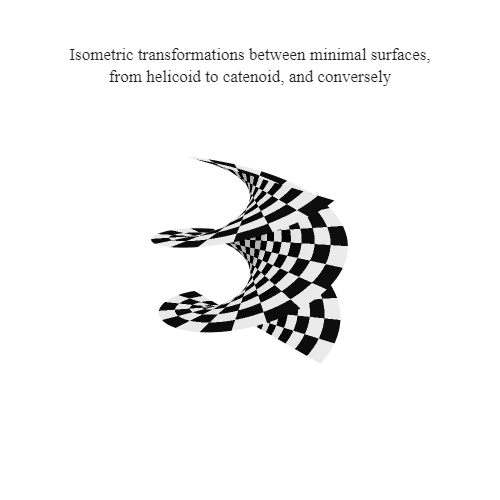# How to define a Matrix{UInt8} exhibiting chess board patterns

I need a `Matrix{UInt8}`, P, of 0 and 1 which exhibits chess board patterns
of submatrices A of size(A)=(5,5).
I defined it as follows:

``````P=zeros(UInt8, 100,100)
A= fill(UInt8(1), (5,5))
for k in 0:2:18
for j in 0:2:18
P[5k+1:5k+5, 5j+1:5j+5] .=A
end
end
for k in 1:2:19
for j in 1:2:19
P[5k+1:5k+5, 5j+1:5j+5] .=A
end
end
``````

Is there a simpler method to define P?

Simpler? Maybe, you can for instance just replace `A` with `UInt8(1)`. Shorter? Absolutely:

``````P = [UInt8(mod(div(i, 5) + div(j, 5), 2)) for i in 1:100, j in 1:100]
``````

(I’m writing this on my phone after a quite wet midsummer celebration, so I don’t guarantee it works. If you want me to test it, ping me tomorrow)

With a slight modification P is defined as:

``````P=[UInt8(mod(div(i+4, 5)+div(j+4, 5), 2)) for i=1:100, j=1:100]
``````

Thank you!!!

For this specific example there is also the `repeat` function.

1 Like
``````
Z(s)=zeros(Int, s,s)
O(s)=ones(Int, s,s)
blockm(s,m,n)=@view hvcat(ntuple(_->n ÷ 2+n%2  ,m÷2+m%2),fill([Z(s) O(s) ; O(s) Z(s)], n ÷ 2+n%2  ,m÷ 2+m%2)...)[1:s*m,1:s*n]

julia> blockm(3,4,3)
12×9 view(::Matrix{Int64}, 1:12, 1:9) with eltype Int64:
0  0  0  1  1  1  0  0  0
0  0  0  1  1  1  0  0  0
0  0  0  1  1  1  0  0  0
1  1  1  0  0  0  1  1  1
1  1  1  0  0  0  1  1  1
1  1  1  0  0  0  1  1  1
0  0  0  1  1  1  0  0  0
0  0  0  1  1  1  0  0  0
0  0  0  1  1  1  0  0  0
1  1  1  0  0  0  1  1  1
1  1  1  0  0  0  1  1  1
1  1  1  0  0  0  1  1  1
``````

in the solution expression the indices should start from 0 not from 1. isn’t it?

Yes, but as I mentioned above I modified @gustaphe’s i and j.

like this or is there a more direct way?

``````blockm(s,m,n) = repeat([0 1; 1 0],inner=(s,s), outer=((m÷2)+(m%2),(n÷2)+(n%2)))[1:m*s,1:n*s]
``````

Though not memory efficient, you could do it easily with the Kronecker Product.
Create a regular chess pattern and multiply it by a matrix of ones of the size of the block you want.

simil kroneker

``````O(s)=ones(Bool,s,s)
mb(n,m)=[(i+j)%2==0 for i in 1:n, j in 1:m]

mb(m,n)=reduce(hcat,accumulate(xor, fill(true,m),init=i) for i in accumulate(xor,fill(true,n)))

blockm(s,m,n)=hvcat(ntuple(_->n,m),mb(n,m).*[O(s)]...)
``````
``````O(s)=ones(Bool,s,s
b(x) = accumulate(xor,fill(true,x))
blockm(s,m,n) = hvcat(ntuple(_->n,m),xor.(b(n), b(m)').*[O(s)]...)
``````1 Like

Simple enough to fix, just add 1 to the argument of `mod`. I thought demonstrating the principle was meaningful even without spending too long parsing the example.

It wasn’t meant to be a critical remark, just a request for clarification to make sure I understood the problem.

is the table the initial condition of this transformation?
what is the code that produces this animation?

The posted animation contains 140 frames. This is the code for the first 35 frames:

``````using Plots
plotlyjs();

function intermsurf(;r=2,  α=0.0)
n = 100
v = range(-r, r; length = n)
u = range(-π, π; length = n)
x = @. cos(α)*sinh(v)*sin(u)' + sin(α)*cosh(v)*cos(u)'
y = @. sin(α)*cosh(v)*sin(u)' - cos(α)*sinh(v)*cos.(u)'
z = sin(α)*collect(v)*ones(size(u))' +cos(α)*ones(size(v))*collect(u)'
return x, y, z
end
bw = cgrad([:black, RGB{Float64}(0.9, 0.9, 0.9)])
surfpatterns = [UInt8(mod(div(i+4,5)+div(j+4,5), 2)) for i=1:100, j=1:100];

a=vcat(collect(range(0, π/2; length=30)), fill(π/2, 5))
@gif for α∈a
x, y, z= intermsurf(; α=α)
Plots.surface(x, y, z, fill_z = surfpatterns, color=palette(bw, 2),
colorbar=false, showaxis=false,
size=(450, 400), camera=(45, 20))
end
``````